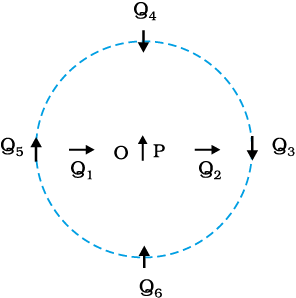Select Chapter Topics:Which of the following statement is incorrect?

 1 If a bar magnet is cut into two pieces transverse to its length and along its length, in either case, one gets two magnets, each with a north and south pole. 2 A magnetized needle in a uniform magnetic field experiences a torque but no net force. An iron nail near a bar magnet, however, experiences a force of attraction in addition to torque. 3 Every magnetic configuration must have a north pole and a south pole. 4 In a bar magnet, the intensity of the magnetic field is the strongest at the two ends (poles) and weakest at the central region.Subtopic:  Bar Magnet |
To view explanation, please take trial in the course.
NEET 2023 - Target Batch - Aryan Raj Singh
Hints

In the figure, the magnetic needle has a magnetic moment of 6.7 × 10–2 Am2 and a moment of inertia I = 7.5 × 10–6 kg m2. It performs 10 complete oscillations in 6.70 s in a magnetic field. What is the magnitude of the magnetic field?1. 0.02 T

2. 0.01 T

3. 003 T

4. 0.001 TSubtopic:  Bar Magnet |
59%
To view explanation, please take trial in the course.
NEET 2023 - Target Batch - Aryan Raj Singh
Hints

A short bar magnet placed with its axis at 30° with an external field of 800 G experiences a torque of 0.016 Nm. What is the magnetic moment of the magnet?Subtopic:  Bar Magnet |
81%
To view explanation, please take trial in the course.
NEET 2023 - Target Batch - Aryan Raj Singh
Hints

A short bar magnet placed with its axis at 30° with an external field of 800 G experiences a torque of 0.016 N-m. What is the work done in moving it from its most stable to the most unstable position?

1. 0.036 J

2. 0.016 J

3. 0.064 J

4. 0Subtopic:  Bar Magnet |
71%
To view explanation, please take trial in the course.
NEET 2023 - Target Batch - Aryan Raj Singh
Hints
To view explanation, please take trial in the course.
NEET 2023 - Target Batch - Aryan Raj Singh

A solenoid of cross-sectional area $$2\times 10^{-4}~\mathrm{m^2}$$ and $$1000$$ turns placed with its axis at $$30^\circ$$ with an external field of $$800$$ G experiences a torque of $$0.016$$ Nm. The current flowing through the solenoid is:
1. $$2~\mathrm{A}$$
2. $$4~\mathrm{A}$$
3. $$1~\mathrm{A}$$
4.
$$5~\mathrm{A}$$Subtopic:  Bar Magnet |
63%
To view explanation, please take trial in the course.
NEET 2023 - Target Batch - Aryan Raj Singh
Hints
To view explanation, please take trial in the course.
NEET 2023 - Target Batch - Aryan Raj Singh

The ratio of the magnitudes of the equatorial and axial fields due to a bar magnet of length 5.0 cm at a distance of 50 cm from its mid-point is:
(Given, the magnetic moment of the bar magnet is $$0.40~Am^{2}.$$${}^{}$)
1. $$\frac{1}{2}$$
2. $$2$$
3. $$1$$
4.
$$\frac{3}{2}$$Subtopic:  Analogy between Electrostatics & Magnetostatics |
72%
From NCERT
To view explanation, please take trial in the course.
NEET 2023 - Target Batch - Aryan Raj Singh
Hints
To view explanation, please take trial in the course.
NEET 2023 - Target Batch - Aryan Raj Singh

The figure shows a small magnetized needle P placed at a point O. The arrow shows the direction of its magnetic moment. The other arrows show different positions (and orientations of the magnetic moment) of another identical magnetized needle Q. Then:1 In  configuration, the system is not in equilibrium. 2 In configuration, the system is unstable. 3 In  configuration, the system is stable. 4 $P{Q}_{5}$ configuration corresponds to the lowest potential energy among all the configurations shown.Subtopic:  Analogy between Electrostatics & Magnetostatics |
50%
To view explanation, please take trial in the course.
NEET 2023 - Target Batch - Aryan Raj Singh
Hints

Which of the following is the correct representation of magnetic field lines?1. (g), (c)

2. (d), (f)

3. (a), (b)

4. (c), (e)Subtopic:  Magnetic Field & Field Lines |
85%
To view explanation, please take trial in the course.
NEET 2023 - Target Batch - Aryan Raj Singh
Hints

Which one of the following is correct?

 1 The magnetic field lines also represent the lines of force on a moving charged particle at every point. 2 Magnetic field lines can be entirely confined within the core of a toroid, but not within a straight solenoid. 3 A bar magnet exerts a torque on itself due to its own field. 4 Magnetic field arises due to stationary charges.Subtopic:  Magnetic Field & Field Lines |
To view explanation, please take trial in the course.
NEET 2023 - Target Batch - Aryan Raj Singh
Hints

A solenoid has a core of material with relative permeability $$400.$$ The windings of the solenoid are insulated from the core and carry a current of $$2$$ A. If the number of turns is $$1000$$ per metre, the magnetic field intensity $$H$$ is:
1. $$2\times10^2$$ A/m
2. $$2\times10^3$$ A/m
3. $$2$$ A/m
4. $$20$$ A/mSubtopic:  Magnetization & Magnetic Intensity |
75%
From NCERT
To view explanation, please take trial in the course.
NEET 2023 - Target Batch - Aryan Raj Singh
Hints